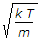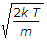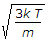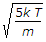# Mechanical Engineering - Thermodynamics

### Exercise :: Thermodynamics - Section 3

31.

According to kinetic theory of gases, the velocity of molecules __________ with the increase in temperature.

 A. remains constant B. increases C. decreases

Explanation:

No answer description available for this question. Let us discuss.

32.

The hard coke is obtained when carbonisation of coal is carried out at

 A. 300° to 500°C B. 500° to700°C C. 700° to 900°C D. 900° to 1100°C

Explanation:

No answer description available for this question. Let us discuss.

33.

According to First law of thermodynamics,

 A. total internal energy of a system during a process remains constant B. total energy of a system remains constant C. workdone by a system is equal to the heat transferred by the system D. internal energy, enthalpy and entropy during a process remains constant

Explanation:

No answer description available for this question. Let us discuss.

34.

The root mean square velocity of the gas molecules is given by (where k = Boltzmann's constant, T = Absolute temperature, and m = Mass of one molecule of a gas)

 A.B.C.D.Explanation:

No answer description available for this question. Let us discuss.

35.

Stirling cycle consists of

 A. two constant volume and two isentropic processes B. two constant volume and two isothermal processes C. two constant pressure and two isothermal processes D. one constant volume, one constant pressure and two isentropic processes